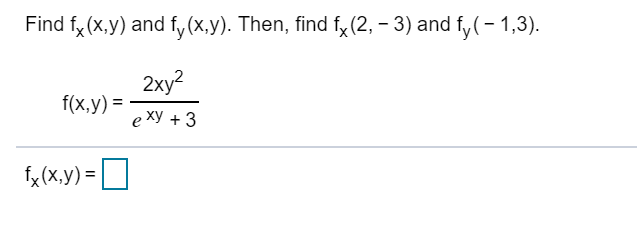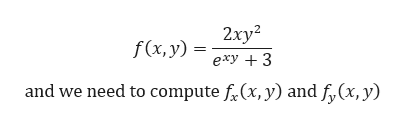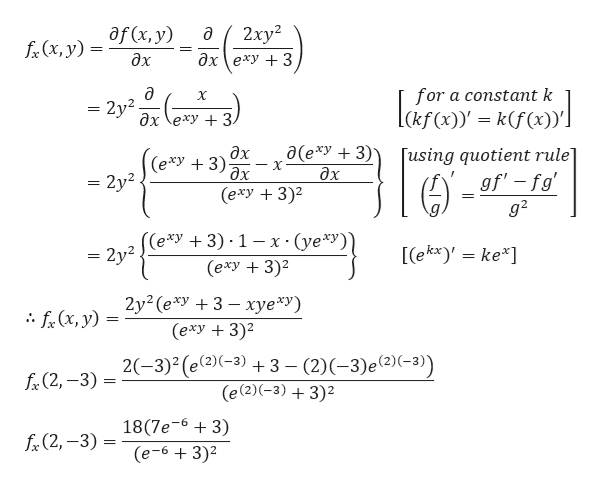# Find f(x,y) and fy(x,y). Then, find fx (2, 3) and fy(-1,3)2ху?f(x,y)e ху + 3f(x.y)=

Question
1 viewshelp_outlineImage TranscriptioncloseFind f(x,y) and fy(x,y). Then, find fx (2, 3) and fy(-1,3) 2ху? f(x,y) e ху + 3 f(x.y)= fullscreen
check_circle

Step 1

Given,help_outlineImage Transcriptionclose2хy? f(x, y) exy 3 and we need to compute f(x, y) and f,(x, y) fullscreen
Step 2

For computing the partial derivative fx(x,y), we wil...help_outlineImage Transcriptionclose2ху? дх (еху + 3 af (x, y) fr(x, y) дх for a constant k ckfx)(f()) a X = 2y2. дх \еху + 3. a(e*y3) дх (еху + 3)2 дх (exy 3) дх Tusing quotient rule] gf'-fg = 2y2 g2 (е*У + 3) 1 — х (yе*У) (exy 3) [ekx)' ke* = 2y2 2y2 (e*y3 xye*y) (exy3) f(x, y) 3 (2)-3)e2)(-3)) (e(2)(-3)3)2 2-3)2 (e(2)(-3) fe(2,-3) = 18(7e-63) fr(2,-3) (e-6+3)2 fullscreen

### Want to see the full answer?

See Solution

#### Want to see this answer and more?

Solutions are written by subject experts who are available 24/7. Questions are typically answered within 1 hour.*

See Solution
*Response times may vary by subject and question.
Tagged in

### Derivative